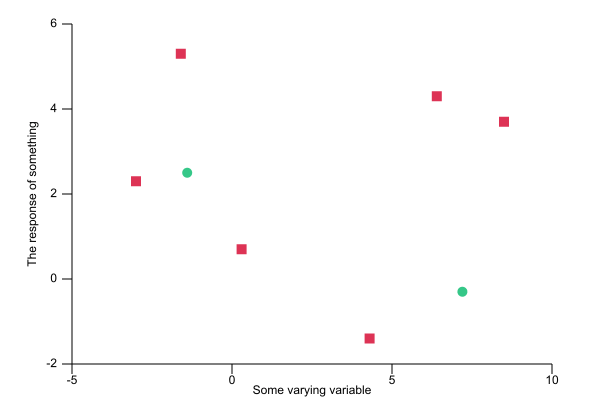## plotlib

Pure Rust plotting library

### 4 releases(breaking)

✓ Uses Rust 2018 edition

 0.4.0 Mar 2, 2019 Mar 1, 2018 Mar 16, 2017 Mar 9, 2017

#7 in Visualization

Used in 2 crates

105KB
3K SLoC

# plotlib`plotlib` is a generic data visualisation and plotting library for Rust. It is currently in the very early stages of development.

It can currently produce:

• histograms
• scatter plots
• line graphs from data or from function definitions
• box plots
• bar charts

rendering them as either SVG or plain text.

The API is still very much in flux and is subject to change.

For example, code like:

``````use plotlib::scatter::Scatter;
use plotlib::scatter;
use plotlib::style::{Marker, Point};
use plotlib::view::View;
use plotlib::page::Page;

fn main() {
// Scatter plots expect a list of pairs
let data1 = [(-3.0, 2.3), (-1.6, 5.3), (0.3, 0.7), (4.3, -1.4), (6.4, 4.3), (8.5, 3.7)];

// We create our scatter plot from the data
let s1 = Scatter::from_slice(&data1)
.style(scatter::Style::new()
.marker(Marker::Square) // setting the marker to be a square
.colour("#DD3355")); // and a custom colour

// We can plot multiple data sets in the same view
let data2 = [(-1.4, 2.5), (7.2, -0.3)];
let s2 = Scatter::from_slice(&data2)
.style(scatter::Style::new() // uses the default marker
.colour("#35C788")); // and a different colour

// The 'view' describes what set of data is drawn
let v = View::new()
.x_range(-5., 10.)
.y_range(-2., 6.)
.x_label("Some varying variable")
.y_label("The response of something");

// A page with a single view is then saved to an SVG file
Page::single(&v).save("scatter.svg");
}
``````

will produce output like:~115KB